# K-system(2)

(diff) ← Older revision | Latest revision (diff) | Newer revision → (diff)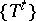A measurable flow (-flow) or cascade (-cascade) in a Lebesgue space such that there is a measurable partition (cf. Measurable decomposition)of the phase space with the following properties: a) it is increasing (formerly called invariant) with respect to, that is,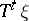is a refinement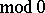ofwhen; b) it is a two-sided generator for, that is, the only measurable partitionthat is finerthan all theis the partition into points; and c) the only measurable partitionthat is coarserthan all theis the trivial partition, whose only element is the whole phase space.

An automorphism of a measure space whose iterations form a-cascade is called a-automorphism. Ifis a-system, then allwith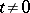are-automorphisms. Conversely, given a measurable flow or cascade, if just one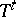is a-automorphism, thenis a-system.-systems posses strong ergodic properties: positive entropy (cf. Entropy theory of a dynamical system) and ergodicity; mixing of all degrees and they have a countably-multiple Lebesgue spectrum (see Spectrum of a dynamical system; and also ).

An endomorphism of a Lebesgue space has completely-positive entropy if all its non-trivial quotient endomorphisms have positive entropy. Among these are the-automorphisms (namely, they are just the automorphisms with completely-positive entropy) and also other interesting objects (exact endomorphisms; cf. Exact endomorphism). The notion of a-system can be generalized in other directions: to the case of an infinite invariant measure (see , , ) and for the action of groups other thanand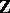(see , ).-systems are sometimes called Kolmogorov systems (flows, etc.), after their originator (see ), who used the term "quasi-regular dynamical systemquasi-regular" . This emphasizes the analogy with regular random processes (see ). If a random process, stationary in the narrow sense of the word, is interpreted as a dynamical system, then the values of the process "in the past" define a certain increasing measurable partition, which is the smallest with respect to which all the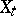with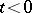are measurable. Ifhas the properties b) and c) above (the "all or nothing" law), then the process is called regular. In particular, this probabilistic form presents the simplest example of a-automorphism: a Bernoulli automorphism.

Given a measurable flow or cascade in a Lebesgue space, if one of theis isomorphic to a Bernoulli automorphism, then they all are (when). In this case the dynamical system is called Bernoullian (see ). There are-systems that are not Bernoullian.-systems (even Bernoullian ones) arise naturally not only in probability theory, but also in problems of an algebraic, geometric and even physical nature (see , , , , ).

How to Cite This Entry:
K-system(2). Encyclopedia of Mathematics. URL: http://encyclopediaofmath.org/index.php?title=K-system(2)&oldid=18048
This article was adapted from an original article by D.V. Anosov (originator), which appeared in Encyclopedia of Mathematics - ISBN 1402006098. See original article